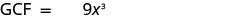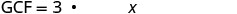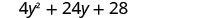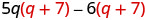# 7.1: Greatest Common Factor and Factor by Grouping

•• OpenStax
• OpenStax
$$\newcommand{\vecs}{\overset { \rightharpoonup} {\mathbf{#1}} }$$ $$\newcommand{\vecd}{\overset{-\!-\!\rightharpoonup}{\vphantom{a}\smash {#1}}}$$$$\newcommand{\id}{\mathrm{id}}$$ $$\newcommand{\Span}{\mathrm{span}}$$ $$\newcommand{\kernel}{\mathrm{null}\,}$$ $$\newcommand{\range}{\mathrm{range}\,}$$ $$\newcommand{\RealPart}{\mathrm{Re}}$$ $$\newcommand{\ImaginaryPart}{\mathrm{Im}}$$ $$\newcommand{\Argument}{\mathrm{Arg}}$$ $$\newcommand{\norm}{\| #1 \|}$$ $$\newcommand{\inner}{\langle #1, #2 \rangle}$$ $$\newcommand{\Span}{\mathrm{span}}$$ $$\newcommand{\id}{\mathrm{id}}$$ $$\newcommand{\Span}{\mathrm{span}}$$ $$\newcommand{\kernel}{\mathrm{null}\,}$$ $$\newcommand{\range}{\mathrm{range}\,}$$ $$\newcommand{\RealPart}{\mathrm{Re}}$$ $$\newcommand{\ImaginaryPart}{\mathrm{Im}}$$ $$\newcommand{\Argument}{\mathrm{Arg}}$$ $$\newcommand{\norm}{\| #1 \|}$$ $$\newcommand{\inner}{\langle #1, #2 \rangle}$$ $$\newcommand{\Span}{\mathrm{span}}$$$$\newcommand{\AA}{\unicode[.8,0]{x212B}}$$

##### Learning Objectives

By the end of this section, you will be able to:

• Find the greatest common factor of two or more expressions
• Factor the greatest common factor from a polynomial
• Factor by grouping
##### BE PREPARED

Before you get started, take this readiness quiz.

1. Factor 56 into primes.
If you missed this problem, review Exercise 1.2.19.
2. Find the least common multiple of 18 and 24.
If you missed this problem, review Exercise 1.2.28.
3. Simplify $$−3(6a+11)$$.
If you missed this problem, review Exercise 1.10.40.

## Find the Greatest Common Factor of Two or More Expressions

Earlier we multiplied factors together to get a product. Now, we will be reversing this process; we will start with a product and then break it down into its factors. Splitting a product into factors is called factoring.We have learned how to factor numbers to find the least common multiple (LCM) of two or more numbers. Now we will factor expressions and find the greatest common factor of two or more expressions. The method we use is similar to what we used to find the LCM.

##### GREATEST COMMON FACTOR

The greatest common factor (GCF) of two or more expressions is the largest expression that is a factor of all the expressions.

First we’ll find the GCF of two numbers.

##### Exercise $$\PageIndex{1}$$: HOW TO FIND THE GREATEST COMMON FACTOR OF TWO OR MORE EXPRESSIONS

Find the GCF of 54 and 36.Notice that, because the GCF is a factor of both numbers, 54 and 36 can be written as multiples of 18.

$\begin{array}{l}{54=18 \cdot 3} \\ {36=18 \cdot 2}\end{array}$

##### Exercise $$\PageIndex{2}$$

Find the GCF of 48 and 80.

16

##### Exercise $$\PageIndex{3}$$

Find the GCF of 18 and 40.

2

We summarize the steps we use to find the GCF below.

##### HOW TO

Find the Greatest Common Factor (GCF) of two expressions.

1. Step 1. Factor each coefficient into primes. Write all variables with exponents in expanded form.
2. Step 2. List all factors—matching common factors in a column. In each column, circle the common factors.
3. Step 3. Bring down the common factors that all expressions share.
4. Step 4. Multiply the factors.

In the first example, the GCF was a constant. In the next two examples, we will get variables in the greatest common factor.

##### Exercise $$\PageIndex{4}$$

Find the greatest common factor of $$27x^3$$ and $$18x^4$$.

 Factor each coefficient into primes and write the variables with exponents in expanded form. Circle the common factors in each column.Bring down the common factors.Multiply the factors.The GCF of 27$$x^{3}$$ and 18$$x^{4}$$ is 9$$x^{3}$$.
##### Exercise $$\PageIndex{5}$$

Find the GCF: $$12 x^{2}, 18 x^{3}$$

$$6x^2$$

##### Exercise $$\PageIndex{6}$$

Find the GCF: $$16 y^{2}, 24 y^{3}$$

$$8y^2$$

##### Exercise $$\PageIndex{7}$$

Find the GCF of $$4 x^{2} y, 6 x y^{3}$$

 Factor each coefficient into primes and write the variables with exponents in expanded form. Circle the common factors in each column.Bring down the common factors.Multiply the factors.The GCF of 4$$x^{2} y$$ and 6$$x y^{3}$$ is 2$$x y .$$
##### Exercise $$\PageIndex{8}$$

Find the GCF: $$6 a b^{4}, 8 a^{2} b$$

$$2ab$$

##### Exercise $$\PageIndex{9}$$

Find the GCF: $$9 m^{5} n^{2}, 12 m^{3} n$$

$$3m^3 n$$

##### Exercise $$\PageIndex{10}$$

Find the GCF of: $$21 x^{3}, 9 x^{2}, 15 x$$

 Factor each coefficient into primes and write the variables with exponents in expanded form. Circle the common factors in each column.Bring down the common factors.Multiply the factors.The GCF of $$21 x^{3}, 9 x^{2}$$ and 15$$x$$ is 3$$x$$
##### Exercise $$\PageIndex{11}$$

Find the greatest common factor: $$25 m^{4}, 35 m^{3}, 20 m^{2}$$

$$5m^2$$

##### Exercise $$\PageIndex{12}$$

Find the greatest common factor: $$14 x^{3}, 70 x^{2}, 105 x$$

$$7x$$

## Factor the Greatest Common Factor from a Polynomial

Just like in arithmetic, where it is sometimes useful to represent a number in factored form (for example, 12 as 2·6or3·4),2·6or3·4), in algebra, it can be useful to represent a polynomial in factored form. One way to do this is by finding the GCF of all the terms. Remember, we multiply a polynomial by a monomial as follows:

$\begin{array}{cc}{2(x+7)} & {\text { factors }} \\ {2 \cdot x+2 \cdot 7} & { } \\ {2 x+14} & {\text { product }}\end{array}$

Now we will start with a product, like $$2 x+14$$, and end with its factors, 2$$(x+7)$$. To do this we apply the Distributive Property “in reverse.”

We state the Distributive Property here just as you saw it in earlier chapters and “in reverse.”

##### DISTRIBUTIVE PROPERTY

If $$a,b,c$$ are real numbers, then

$a(b+c)=a b+a c \quad\text{ and }\quad a b+a c=a(b+c)$

The form on the left is used to multiply. The form on the right is used to factor.

So how do you use the Distributive Property to factor a polynomial? You just find the GCF of all the terms and write the polynomial as a product!

##### Exercise $$\PageIndex{13}$$: HOW TO FACTOR THE GREATEST COMMON FACTOR FROM A POLYNOMIAL

Factor: $$4 x+12$$##### Exercise $$\PageIndex{14}$$

Factor: $$6 a+24$$

$$6(a+4)$$

##### Exercise $$\PageIndex{15}$$

Factor: $$2 b+14$$

$$2(b+7)$$

##### HOW TO

Factor the greatest common factor from a polynomial.

Step 1. Find the GCF of all the terms of the polynomial.

Step 2. Rewrite each term as a product using the GCF.

Step 3. Use the “reverse” Distributive Property to factor the expression.

Step 4. Check by multiplying the factors.

##### FACTOR AS A NOUN AND A VERB

We use “factor” as both a noun and a verb.##### Exercise $$\PageIndex{16}$$

Factor: $$5 a+5$$

 Find the GCF of 5a and 5.Rewrite each term as a product using the GCF.Use the Distributive Property "in reverse" to factor the GCF.Check by multiplying the factors to get the original polynomial. 5$$(a+1)$$ $$5 \cdot a+5 \cdot 1$$ $$5 a+5 \checkmark$$
##### Exercise $$\PageIndex{17}$$

Factor: $$14 x+14$$

$$14(x+1)$$

##### Exercise $$\PageIndex{18}$$

Factor: $$12 p+12$$

$$12(p+1)$$

The expressions in the next example have several factors in common. Remember to write the GCF as the product of all the common factors.

##### Exercise $$\PageIndex{19}$$

Factor: $$12 x-60$$

 Find the GCF of 12x and 60.Rewrite each term as a product using the GCF.Factor the GCF.Check by multiplying the factors. 12(x−5) $$12 \cdot x-12 \cdot 5$$ $$12 x-60 \checkmark$$
##### Exercise $$\PageIndex{20}$$

Factor: $$18 u-36$$

$$8(u-2)$$

##### Exercise $$\PageIndex{21}$$

Factor: $$30 y-60$$

$$30(y-2)$$

Now we’ll factor the greatest common factor from a trinomial. We start by finding the GCF of all three terms.

##### Exercise $$\PageIndex{22}$$

Factor: $$4 y^{2}+24 y+28$$

We start by finding the GCF of all three terms.

 Find the GCF of $$4 y^{2}, 24 y$$ and 28Rewrite each term as a product using the GCF.Factor the GCF.Check by multiplying. 4$$\left(y^{2}+6 y+7\right)$$ $$4 \cdot y^{2}+4 \cdot 6 y+4 \cdot 7$$ $$4 y^{2}+24 y+28 \checkmark$$
##### Exercise $$\PageIndex{23}$$

Factor: $$5 x^{2}-25 x+15$$

$$5\left(x^{2}-5 x+3\right)$$

##### Exercise $$\PageIndex{24}$$

Factor: $$3 y^{2}-12 y+27$$

$$3\left(y^{2}-4 y+9\right)$$

##### Exercise $$\PageIndex{25}$$

Factor: $$5 x^{3}-25 x^{2}$$

 Find the GCF of 5$$x^{3}$$ and 25$$x^{2}$$Rewrite each term.Factor the GCF.Check. 5$$x^{2}(x-5)$$ $$5 x^{2} \cdot x-5 x^{2} \cdot 5$$ $$5 x^{3}-25 x^{2}\checkmark$$
##### Exercise $$\PageIndex{26}$$

Factor: $$2 x^{3}+12 x^{2}$$

$$2x^2(x+6)$$

##### Exercise $$\PageIndex{27}$$

Factor: $$6 y^{3}-15 y^{2}$$

$$3y^2(2y-5)$$

##### Exercise $$\PageIndex{28}$$

Factor: $$21 x^{3}-9 x^{2}+15 x$$

In a previous example we found the GCF of $$21 x^{3}, 9 x^{2}, 15 x$$ to be 3$$x$$.Rewrite each term using the GCF, 3x.Factor the GCF.Check. 3$$x\left(7 x^{2}-3 x+5\right)$$ $$3 x \cdot 7 x^{2}-3 x \cdot 3 x+3 x \cdot 5$$ $$21 x^{3}-9 x^{2}+15 x\checkmark$$
##### Exercise $$\PageIndex{29}$$

Factor: $$20 x^{3}-10 x^{2}+14 x$$

$$2x(10x^2-5x+7)$$

##### Exercise $$\PageIndex{30}$$

Factor: $$24 y^{3}-12 y^{2}-20 y$$

$$4y(6y^2-3y-5)$$

##### Exercise $$\PageIndex{31}$$

Factor: $$8 m^{3}-12 m^{2} n+20 m n^{2}$$

 Find the GCF of $$8 m^{3}, 12 m^{2} n, 20 m n^{2}$$Rewrite each term.Factor the GCF.Check. 4$$m\left(2 m^{2}-3 m n+5 n^{2}\right)$$ $$4 m \cdot 2 m^{2}-4 m \cdot 3 m n+4 m \cdot 5 n^{2}$$ $$8 m^{3}-12 m^{2} n+20 m n^{2}\checkmark$$
##### Exercise $$\PageIndex{32}$$

Factor: $$9 x y^{2}+6 x^{2} y^{2}+21 y^{3}$$

$$3y^2(3x+2x^2+7y)$$

##### Exercise $$\PageIndex{33}$$

Factor: $$3 p^{3}-6 p^{2} q+9 p q^{3}$$

$$3p(p^2-2pq+3q^2$$

When the leading coefficient is negative, we factor the negative out as part of the GCF.

##### Exercise $$\PageIndex{34}$$

Factor: $$-8 y-24$$

When the leading coefficient is negative, the GCF will be negative.

 Ignoring the signs of the terms, we first find the GCF of 8y and 24 is 8. Since the expression −8y − 24 has a negative leading coefficient, we use −8 as the GCF.Rewrite each term using the GCF.Factor the GCF.Check. $$-8(y+3)$$ $$-8 \cdot y+(-8) \cdot 3$$ $$-8 y-24 \checkmark$$
##### Exercise $$\PageIndex{35}$$

Factor: $$-16 z-64$$

$$-16(z+4)$$

##### Exercise $$\PageIndex{36}$$

Factor: $$-9 y-27$$

$$-9(y+3)$$

##### Exercise $$\PageIndex{37}$$

Factor: $$-6 a^{2}+36 a$$

The leading coefficient is negative, so the GCF will be negative.?

 Since the leading coefficient is negative, the GCF is negative, −6a.Rewrite each term using the GCF.Factor the GCF.Check. $$-6 a(a-6)$$ $$-6 a \cdot a+(-6 a)(-6)$$ $$-6 a^{2}+36 a v$$
##### Exercise $$\PageIndex{38}$$

Factor: $$-4 b^{2}+16 b$$

$$-4b(b-4)$$

##### Exercise $$\PageIndex{39}$$

Factor: $$-7 a^{2}+21 a$$

$$-7a(a-3)$$

##### Exercise $$\PageIndex{40}$$

Factor: $$5 q(q+7)-6(q+7)$$

The GCF is the binomial q+7.Factor the GCF, (q + 7).Check on your own by multiplying.
##### Exercise $$\PageIndex{41}$$

Factor: $$4 m(m+3)-7(m+3)$$

$$(m+3)(4m-7)$$

##### Exercise $$\PageIndex{42}$$

Factor: $$8 n(n-4)+5(n-4)$$

$$(n-4)(8n+5)$$

## Factor by Grouping

When there is no common factor of all the terms of a polynomial, look for a common factor in just some of the terms. When there are four terms, a good way to start is by separating the polynomial into two parts with two terms in each part. Then look for the GCF in each part. If the polynomial can be factored, you will find a common factor emerges from both parts.

(Not all polynomials can be factored. Just like some numbers are prime, some polynomials are prime.)

##### Exercise $$\PageIndex{43}$$

Factor: $$x y+3 y+2 x+6$$##### Exercise $$\PageIndex{44}$$

Factor: $$x y+8 y+3 x+24$$

$$(x+8)(y+3)$$

##### Exercise $$\PageIndex{45}$$

Factor: $$a b+7 b+8 a+56$$

$$(a+7)(b+8)$$

##### HOW TO

Factor by grouping.

Step 1. Group terms with common factors.

Step 2. Factor out the common factor in each group.

Step 3. Factor the common factor from the expression.

Step 4. Check by multiplying the factors.

##### Exercise $$\PageIndex{46}$$

Factor: $$x^{2}+3 x-2 x-6$$

$$\begin{array}{ll}{\text { There is no GCF in all four terms. }} & x^{2}+3 x-2 x-6\\ {\text { Separate into two parts. }} & \underbrace{x^{2}+3 x}\underbrace{-2 x-6} \\ \\ {\text { Factor the GCF from both parts. Be careful }} \\ {\text { with the signs when factoring the GCF from }}& \begin{array}{c}{x(x+3)-2(x+3)} \\ {(x+3)(x-2)}\end{array} \\ {\text { the last two terms. }} \\ \\ \text { Check on your own by multinlying. }\end{array}$$

##### Exercise $$\PageIndex{47}$$

Factor: $$x^{2}+2 x-5 x-10$$

$$(x-5)(x+2)$$

##### Exercise $$\PageIndex{48}$$

Factor: $$y^{2}+4 y-7 y-28$$

$$(y+4)(y-7)$$

##### MEDIA ACCESS ADDITIONAL ONLINE RESOURCES

Access these online resources for additional instruction and practice with greatest common factors (GFCs) and factoring by grouping.

• Greatest Common Factor (GCF)
• Factoring Out the GCF of a Binomial
• Greatest Common Factor (GCF) of Polynomials

## Glossary

factoring
Factoring is splitting a product into factors; in other words, it is the reverse process of multiplying.
greatest common factor
The greatest common factor is the largest expression that is a factor of two or more expressions is the greatest common factor (GCF).

This page titled 7.1: Greatest Common Factor and Factor by Grouping is shared under a CC BY license and was authored, remixed, and/or curated by OpenStax.